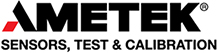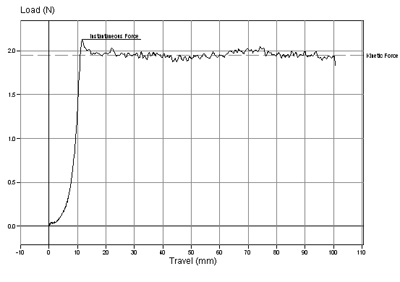Skip to content

# Coefficient of Friction TestingCoefficient of friction (COF) is the maximum value of the frictional force divided by the normal force.

Coefficient of friction (COF) testing is used for a variety of materials from lubricants, to films to household items (ceramic tiles) to determine the frictional characteristics of a material. It is generally determined to be the ease by which two surfaces (often of different materials) slide against each other. It is the maximum value of the frictional force divided by the normal force. The dimensionless value of the COF is the ratio of the force required to slide the surface to the force perpendicular to the surface. A low COF indicates that the surfaces are smoother, e.g. less resistant to a sliding motion. Polymers used for film production are usually tested dry against a sled with a calibrated load.

Common results available for COF are:

Static friction or coefficient
Static friction is the force that holds back a stationary object up to the point that it just starts to move. Thus, the static COF concerns the force restricting the movement of an object that is stationary on a relatively smooth, hard surface. It is calculated by finding the initial peak force required to move the sled and dividing the value by the weight of the sled.

Kinetic friction or coefficient
Once static friction is overcome, kinetic friction follows and is the force holding back regular motion. This kinetic COF concerns the force restricting the movement of an object that is sliding on a relatively smooth, hard surface. It is calculated by finding the average load during the test and dividing this by the weight of the sled, which holds the other material. Please note that the static peak force should not be included in the average force.

Note: Friction is defined as the force between two adjoining surfaces. Slip is the opposite of friction so a high COF gives a low slip factor.

Typical graph showing coefficient of friction test:We provide force measurement instruments and materials testing machines for friction testing.